# Electrical Units and Metric Prefixes

Want create site? Find Free WordPress Themes and plugins.

## Metric Prefixes

Most often measurements made on electric circuits are that of current, voltage, resistance, and power. The base units—ampere, volt, ohm, and watts—are the values most commonly used to measure them. Table 1 lists these basic electrical quantities and the symbols that identify them.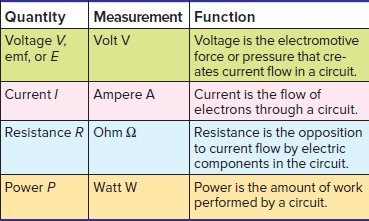Table 1: Electrical Units, Symbols, and Definition

In certain circuit applications the basic electrical units—volt, ampere, ohm, and watt—are either too small or too big to express conveniently. In such cases metric prefixes are often used. Recognizing the meaning of a prefix reduces the possibility of confusion in interpreting data. Common metric prefixes are shown in Table 2.Table 2: Common Metric Prefixes and their Symbols

A metric prefix precedes a unit of measure or its symbol to form decimal multiples or submultiples.

Prefixes are used to reduce the quantity of zeros in numerical equivalencies. For example, in an electrical system, the signal from a sensor may have strength of 0.00125 V, while the voltage applied to the input of a distribution transformer may be in the 27,000-V range. With prefixes, these values would be expressed as 1.25 mV (millivolts) and 27 kV (kilovolts), respectively. Figure 1 shows examples of prefixes used in the rating of electric components.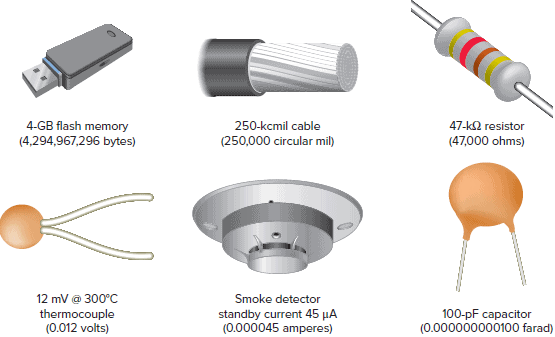Figure 1 Prefixes used in the rating of electric components.

Knowing how to convert metric prefixes back to base units is needed when reading digital multimeters or using electric circuit formulas. Figure 2 and the following examples illustrate how many positions the decimal point is moved to get from a base unit to a multiple or a submultiple of the base unit.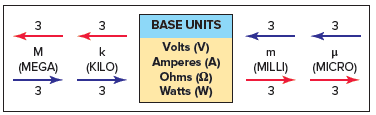Figure 2 Movement of the decimal point to and from base units.

EXAMPLE 1

To convert amperes (A) to milliamperes (mA), it is necessary to move the decimal point three places to the right (this is the same as multiplying the number by 1,000).EXAMPLE 2

To convert milliamperes (mA) to amperes (A), it is necessary to move the decimal point three places to the left (this is the same as multiplying by 0.001).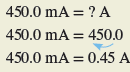EXAMPLE 3

To convert volts (V) to kilovolts (kV), it is necessary to move the decimal point three places to the left.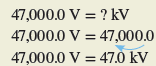EXAMPLE 4

To convert from megohms (MΩ) to ohms (Ω), it is necessary to move the decimal point six places to the right.EXAMPLE 5

To convert from microamperes (μA) to amperes (A), it is necessary to move the decimal point six places to the left.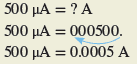Review Questions

1. What is the base unit and symbol used for electric:
1. Current
2. Voltage
3. Resistance
4. Power
2. Write the metric prefix and symbol used to represent each of the following:
1. One-thousandth
2. One million
3. One millionth
4. One thousand
3. Convert each of the following:
1. 2,500 Ω to kilohms
2. 120 kΩ to ohms
3. 1,500,000 Ω to megohms
4. 2.03 MΩ to ohms
5. 0.000466 A to micro-amps
6. 0.000466 A to milliamps
7. 378 mV to volts
8. 475 Ω to kilohms
9. 28 μA to amps
10. 5 kΩ + 850 Ω to kilohms
11. 40,000 kV to megavolts
12. 4,600,000 μA to amps
13. 2.2 kΩ to ohms